# Measuring Distances in Astronomy with GeometryPage 1

#### WATCH ALL SLIDES

Slide 1MEASURING DISTANCES IN ASTRONOMY

Basic Principles:

Geometric methods

Standard candles

Standard rulers

[the last two methods relate quantities that are independent of distance to quantities that depend on distance]

Slide 2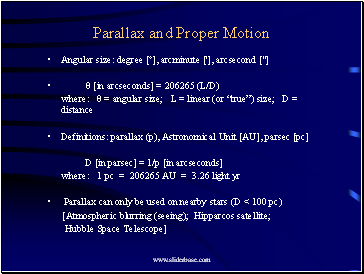## Parallax and Proper Motion

Angular size: degree [º], arcminute ['], arcsecond ["]

θ [in arcseconds] = 206265 (L/D)

where: θ = angular size; L = linear (or “true”) size; D = distance

Definitions: parallax (p), Astronomical Unit [AU], parsec [pc]

D [in parsec] = 1/p [in arcseconds]

where: 1 pc = 206265 AU = 3.26 light yr

Parallax can only be used on nearby stars (D < 100 pc)

[Atmospheric blurring (seeing); Hipparcos satellite;

Hubble Space Telescope]

Slide 3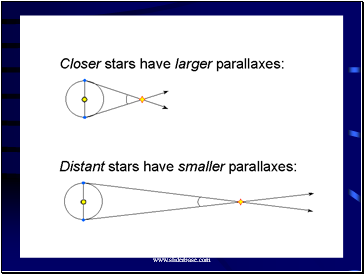Slide 4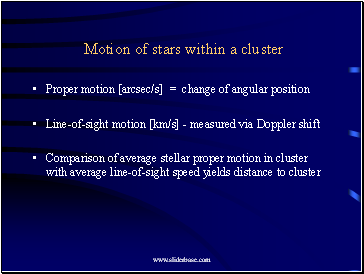## Motion of stars within a cluster

Proper motion [arcsec/s] = change of angular position

Line-of-sight motion [km/s] - measured via Doppler shift

Comparison of average stellar proper motion in cluster with average line-of-sight speed yields distance to cluster

Slide 5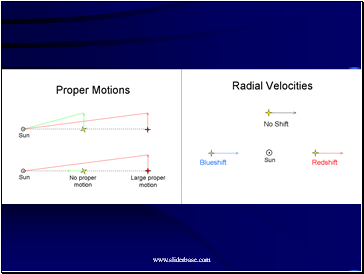Slide 6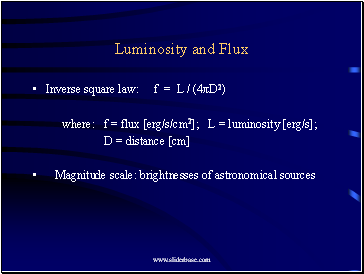## Luminosity and Flux

Inverse square law: f = L / (4πD2)

where: f = flux [erg/s/cm2]; L = luminosity [erg/s];

D = distance [cm]

Magnitude scale: brightnesses of astronomical sources

Slide 7## Standard Candles and Rulers

Variable stars: Cepheids and RR Lyrae stars

Period-luminosity relation; measure P & infer L; measure f & infer D

Other standard candles: brightest red giants, HII regions,

planetary nebulae, supernovae, globular cluster luminosity

Galaxies: Luminosity is seen to be correlated with the typical speed of internal motion of stars and gas

[Tully-Fisher relation: rotation of disks of spiral galaxies]

[Faber-Jackson relation: random stellar motion in elliptical galaxies]

Galaxies: Size correlated with typical speed of (random) stellar motion

[Dn-σ relation for elliptical galaxies]

Slide 8## Redshift as Distance Indicator

Expansion of the Universe

Hubble's law: v = H0 D

where: H0 = Hubble constant [km/s/Mpc]

Doppler shift used to measure recession velocity:

v ≈ c (Δλ / λ)

where: Δλ/λ = fractional change in wavelength

Go to page:
1  2  3